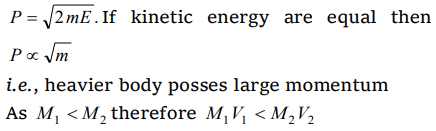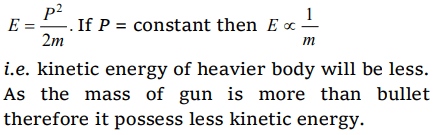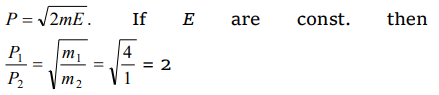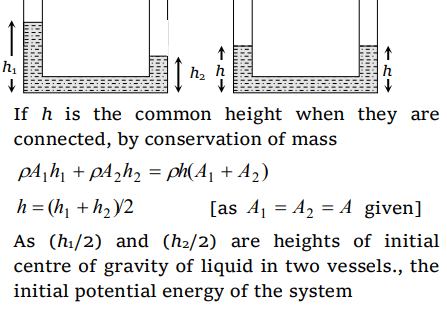## Work, Energy and Power Questions and Answers Part-10

1. In which case does the potential energy decrease
a) On compressing a spring
b) On stretching a spring
c) On moving a body against gravitational force
d) On the rising of an air bubble in water

Explanation: In compression or extension of a spring work is done against restoring force. In moving a body against gravity work is done against gravitational force of attraction. It means in all three cases potential energy of the system increases. But when the bubble rises in the direction of upthrust force then system works so the potential energy of the system decreases.

2. Two bodies of masses m and 2m have same momentum. Their respective kinetic energies $E_{1}$ and $E_{2}$ are in the ratio
a) 1 : 2
b) 2 : 1
c) $1:\sqrt{2}$
d) 1 : 4

Explanation:3.If a lighter body (mass $M_{1}$ and velocity $V_{1}$ ) and a heavier body (mass $M_{2}$ and velocity $V_{2}$ ) have the same kinetic energy, then
a) $M_{2}V_{2}< M_{1}V_{1}$
b) $M_{2}V_{2}= M_{1}V_{1}$
c) $M_{2}V_{1}= M_{1}V_{2}$
d) $M_{2}V_{2}>M_{1}V_{1}$

Explanation:4. If the kinetic energy of a body becomes four times of its initial value, then new momentum will
a) Becomes twice its initial value
b) Become three times its initial value
c) Become four times its initial value
d) Remains constant

Explanation:5.A bullet is fired from a rifle. If the rifle recoils freely, then the kinetic energy of the rifle is
a) Less than that of the bullet
b) More than that of the bullet
c) Same as that of the bullet
d) Equal or less than that of the bullet

Explanation:6. If the water falls from a dam into a turbine wheel 19.6 m below, then the velocity of water at the turbine is$\left(g=9.8m\diagup s^{2}\right)$
a) 9.8 m/s
b) 19.6 m/s
c) 39.2 m/s
d) 98.0 m/s

Explanation:7. Two bodies of masses 2m and m have their K.E. in the ratio 8 : 1, then their ratio of momenta is
a) 1 : 1
b) 2 : 1
c) 4 : 1
d) 8 : 1

Explanation:8. A bomb of 12 kg divides in two parts whose ratio of masses is 1 : 3. If kinetic energy of smaller part is 216 J, then momentum of bigger part in kg-m/ sec will be
a) 36
b) 72
c) 108
d) Data is incomplete

Explanation:9.A 4 kg mass and a 1 kg mass are moving with equal kinetic energies. The ratio of the magnitudes of their linear momenta is
a) 1 : 2
b) 1 : 1
c) 2 : 1
d) 4 : 110. Two identical cylindrical vessels with their bases at same level each contains a liquid of density $\rho$.The height of the liquid in one vessel is $h_{1}$ and that in the other vessel is $h_{2}$ . The area of either base is A. The work done by gravity in equalizing the levels when the two vessels are connected, is
a) $\left(h_{1}-h_{2}\right)g\rho$
b) $\left(h_{1}-h_{2}\right)gA\rho$
c) $\frac{1}{2}\left(h_{1}-h_{2}\right)^2gA\rho$
d) $\frac{1}{4}\left(h_{1}-h_{2}\right)^2gA\rho$